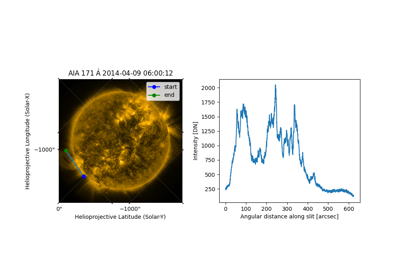# extract_along_coord¶

sunpy.map.extract_along_coord(smap, coord)[source]

Extract pixel values from a map along a path that approximates a coordinate path.

Each pair of consecutive coordinates in the provided coordinate array defines a line segment in pixel space. The approximate pixel path for each line segment is determined by applying Bresenham’s line algorithm. The resulting pixel path does not necessarily include every pixel that is intersected by each line segment.

Parameters:
Returns:

• pixel_values (`Quantity`) – The pixel values along a path that approximates the coordinate path.

• pixel_coords (`SkyCoord`) – The coordinates for the pixels from which the values were extracted.

Notes

The provided coordinates are first rounded to the nearest corresponding pixel, which means that the coordinates used for calculations may be shifted relative to the provided coordinates by up to half a pixel.

ExamplesExtracting intensity of a map along a line

Extracting intensity of a map along a line